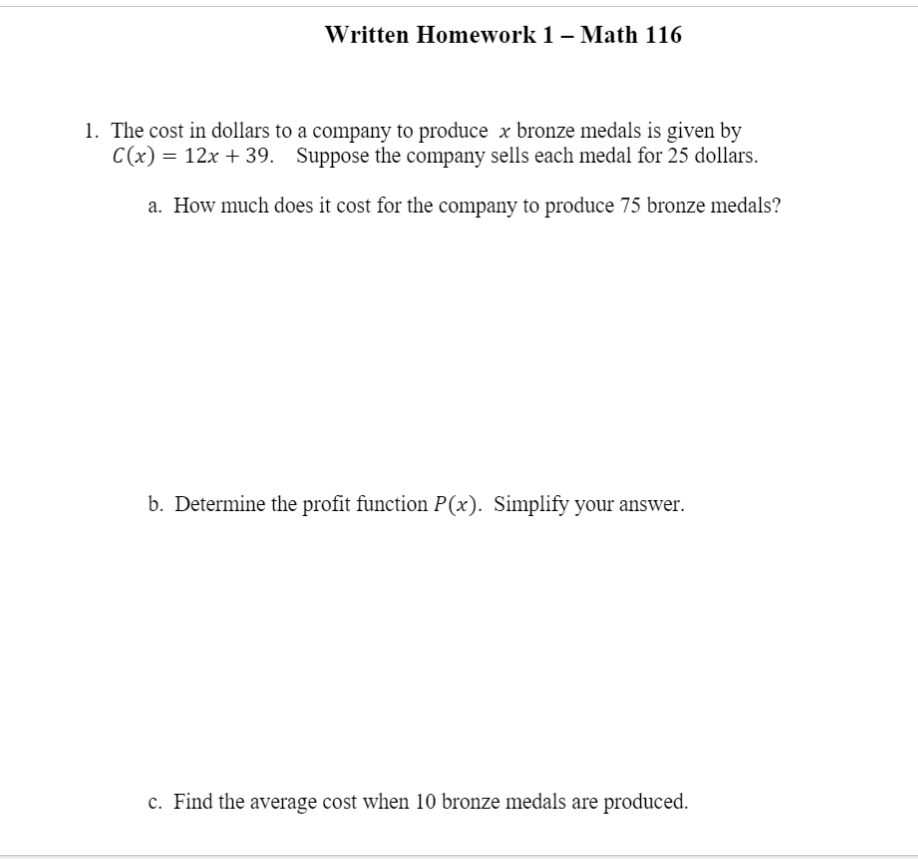# Question Solved1 AnswerWritten Homework 1 - Math 116 1. The cost in dollars to a company to produce x bronze medals is given by C(x) = 12x + 39. Suppose the company sells each medal for 25 dollars. a. How much does it cost for the company to produce 75 bronze medals? b. Determine the profit function P(x). Simplify your answer. c. Find the average cost when 10 bronze medals are produced.Transcribed Image Text: Written Homework 1 - Math 116 1. The cost in dollars to a company to produce x bronze medals is given by C(x) = 12x + 39. Suppose the company sells each medal for 25 dollars. a. How much does it cost for the company to produce 75 bronze medals? b. Determine the profit function P(x). Simplify your answer. c. Find the average cost when 10 bronze medals are produced.
More
Transcribed Image Text: Written Homework 1 - Math 116 1. The cost in dollars to a company to produce x bronze medals is given by C(x) = 12x + 39. Suppose the company sells each medal for 25 dollars. a. How much does it cost for the company to produce 75 bronze medals? b. Determine the profit function P(x). Simplify your answer. c. Find the average cost when 10 bronze medals are produced.